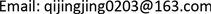1. 引言

2. 基于银企间多层金融网络的模型构建2.1. 银企间网络模型的构成要素

A j i , γ = { a j i , γ } N * N ( M ) 来表示各节点之间的关系， γ 表示不同层：银行间同业拆借层(第1层)、银企间借贷层

(第2层)、企业担保层(第3层)； a j i , γ 表示第 γ 层上的第i个节点拥有第j个节点的债权金额，相当于i的风险敞口，按惯例方向是从节点j指向节点i；统一定义 a i i , γ = 0 表示不存在自己对自己的债权。

2.2. 银行和企业两类主体的微观结构

Simplified bank balance shee

Simplified firm balance shee

3. 基于银企间多层金融网络的风险传导机制3.1. 简化的风险传导机制

3.2. 银行同业拆借层的风险传导机制

3.3. 银企间借贷层的风险传导机制

3.4. 企业担保层的风险传导机制

3.5. 分步骤的风险传导机制

f5多次受到风险的传导。

4. 基于银企间多层金融网络的模型思路分析4.1. 对风险状态的测度思路

4.2. 基于Debtrank算法的风险测度模型构建

H i ( t ) = min { 1 , H i ( t − 1 ) + ∑ C j ( t − 1 ) = D W j i H j ( t − 1 ) } (1)

S i ( t ) = { D , H i ( t ) > 0 ; S i ( t − 1 ) ≠ I I , S i ( t − 1 ) = D S i ( t − 1 ) , otherwise (2)

Debtrank算法是一种受反馈中心性启发的新颖度量方法，能够量化在外部冲击下，特定节点产生财务困境并将风险扩散至整个网络的程度。Battiston等(2012)  将整个网络归一化，定义整个市场的总资

L O f = m V O F ( 0 ) + ∑ E b ( t − 1 ) ≤ 0 a f b ( t ) + a k f , 3 ( t ) + 1 − q q V O F ( t ) (3)

E f ( t ) = V L F ( t ) + V O F ( t ) − ∑ b = 1 N a f b − L O F ( t ) − L O f (4)

W k f , 3 ( t ) = { 1 q a k f , 3 ( 0 ) E f ( 0 ) , E k ( t − 1 ) > 0 0 , E k ( t − 1 ) ≤ 0 (5)

H f ( t + 1 ) = min { 1 , H f ( t ) + ∑ k = 1 M W k f , 3 [ H k ( t ) − H k ( t − 1 ) ] } (6)

S f ( t ) = { U , H f ( t ) = 0 D , H f ( t ) ∈ ( 0 , 1 ) ∩ S f ( t − 1 ) = { U , D } I , H f ( t ) = 1 ∩ S f ( t − 1 ) = { U , D } (7)

v f = ∑ k a k f , 3 ∑ f ∑ k a k f , 3 (8)

R f = ∑ f H f ( T ) v f − ∑ f H f ( 1 ) v f (9)

a j b ( t + 1 ) = { a j b , γ ( t ) E j ( t ) E j ( t − 1 ) , E j ( t − 1 ) > 0 a j b ( t ) = 0 , E j ( t − 1 ) ≤ 0 (10)

a f b ( t + 1 ) = { a f b ( t ) E f ( t ) E f ( t − 1 ) , E b ( t − 1 ) ≥ φ 3 V b ( 0 ) a f b ( t ) , E b ( t − 1 ) < φ 3 V b ( 0 ) (11)

L O b = 1 − q q { m [ V O B ( 0 ) + a f b ( 0 ) ] + ∑ E j ( t − 1 ) ≤ 0 a j b ( t ) } (12)

E b ( t ) = ∑ j = 1 N a j b ( t ) + ∑ f = 1 M a f b ( t ) + V O B ( t ) − L J B ( t ) − L O B ( t ) − L O b (13)

W j b , γ ( t ) = { 1 q a j b , γ ( 0 ) E b ( 0 ) , E j ( t − 1 ) > 0 0 , E j ( t − 1 ) ≤ 0 (14)

H b , 1 ( t + 1 ) = min { 1 , H b , 1 ( t ) + ∑ j = 1 N W j b [ H j ( t ) − H j ( t − 1 ) ] } (15)

H b , 2 ( t + 1 ) = min { 1 , H b , 2 ( t ) + ∑ f = 1 M W f b [ H f ( t ) − H f ( t − 1 ) ] } (16)

S b ( t ) = { U , H b ( t ) = 0 D , H b ( t ) ∈ ( 0 , 1 ) ∩ S b ( t − 1 ) = { U , D } I , H b ( t ) = 1 ∩ S b ( t − 1 ) = { U , D } (17)

v b = ∑ j a j b , γ ∑ b ∑ j a j b , γ (18)

R b = ∑ b H b ( T ) v b − ∑ b H b ( 1 ) v b (19)

5. 结论